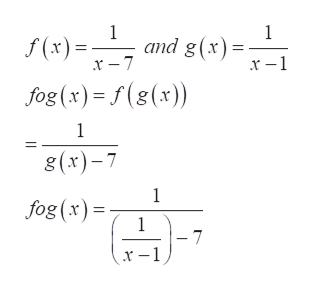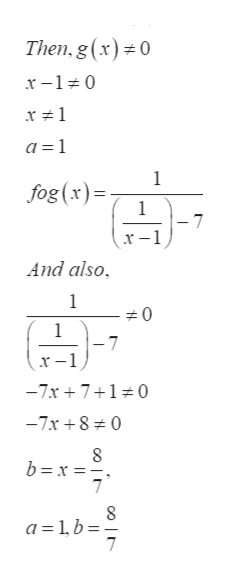# f(x)=1/x-7 and g(x)=1/x-1 then the domain of f o g is equal to all reals except for two values , a and  b with a less than b a=b=

Question
15 views

f(x)=1/x-7 and g(x)=1/x-1 then the domain of f o g is equal to all reals except for two values , a and  b with a less than b

a=

b=

check_circle

Step 1

Refer to the question we have to find the domain of (f o g)(x) is equal to all real values except for two values, a and b with a less than b for the provided functions f(x)=1/x-7 and g(x)=1/x-1.

Step 2

Now to the find the composite function (f o g)(x), it is equal to f(g(x)) then put the value of g(x) for the x in the f(x) as,help_outlineImage Transcriptionclose1 1 f(x) and g(x) fog(x) f(g(x)) 1 8(x)-7 1 fog (x) 1 - 7 x-1) fullscreen
Step 3

Now the domain of the function is defined as that v...help_outlineImage TranscriptioncloseThen, g (x)0 x-10 a 1 1 fog(x)= 1 -7 And also 1 0 1 -7 _ x-1 -7x710 -7x8 0 8 b x 7 a=1b 7 fullscreen

### Want to see the full answer?

See Solution

#### Want to see this answer and more?

Solutions are written by subject experts who are available 24/7. Questions are typically answered within 1 hour.*

See Solution
*Response times may vary by subject and question.
Tagged in

### Other